﻿ 基于相空间重构的混合神经网络对金融混沌序列预测研究

基于相空间重构的混合神经网络对金融混沌序列预测研究Chaotic Financial Series Prediction Study Based on Hybrid Model with Neural Network and Reconstructing Phase Space

Abstract: Strange attractor of Shanghai Stock Index series from 2010 to 2019 is reconstructed by the method of reconstructing phase space, then shows that the largest exponent of Lyapunov is positive through numerical simulation, which confirms that the index series is chaotic. The optimum embedding dimensionality is selected by method of false neighbors. On this basis, a hybrid prediction model based on neural network with genetic algorithm and local forecasting with Gaussian Kernel is proposed, which can forecast chaotic series by estimating the trajectory of strange attractor. Compared with RNN, this prediction model has better convergence speed and prediction accuracy.

1. 引言

2. 文献综述

3. 数据来源及处理

${X}_{i}=\mathrm{ln}{S}_{i}-\mathrm{ln}CP{I}_{i}$ (1)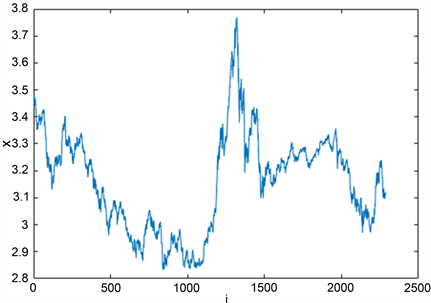Figure 1. Series of modified Shanghai composite index

4. 相空间重构与最大Lyapunov指数

4.1. 相空间重构

$W\left(k,m,\tau \right)=E\left(\underset{|i-j|\ge k}{\sum }\mathrm{ln}\frac{‖{Y}_{i+k}-{Y}_{j+k}‖}{‖{Y}_{i}-{Y}_{j}‖}\right)$ (2)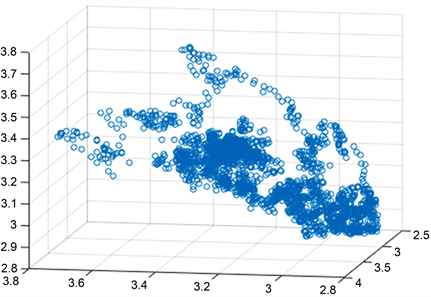Figure 2. Phase space when $m=3$, $\tau =45$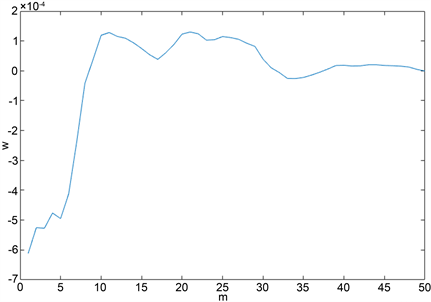Figure 3. Determining the embedding dimension by flase neighbor

4.2. 最大Lyapunov指数

Lyapunov指数是描述混沌系统特征的一个主要参数，在重构的相空间中表示两个相矢随时间演化后的发散程度，即系统对初始条件的敏感程度。负Lyapunov指数代表系统受扰动后的恢复时间，正Lyapunov指数代表系统发散速度，想要知道系统是否混沌的，只需计算最大的Lyapunov指数，大于零即混沌。

$LE=\frac{1}{t}\underset{i=1}{\overset{N}{\sum }}\mathrm{ln}\frac{{l}^{\prime }\left({t}_{i}\right)}{l\left({t}_{i}\right)}$ (3)

t是穷尽整个序列后的总迭代次数。

5. 预测算法

5.1. 遗传算法优化的BP神经网络

1) 虚假邻域法确定神经网络输入节点数；

2) 随机取初始权值；

3) 采用BP算法对所有神经网络权值进行学习；

4) 选取均方误差小的网络结构直接遗传至下一代；

5) 对剩余网络随机进行交叉、变异，产生子网络作为下一代网络结构，检验是否达到设定的均方误差要求；

6) 重复(2)至(5)直到有神经网络的均方误差达到迭代终止要求。

5.2. 基于高斯核权函数的局部预测算子

$K\left(u\right)=\frac{1}{{\left(2\pi \right)}^{m/2}{|\Sigma |}^{1/2}}\mathrm{exp}\left(-\frac{1}{2}u{\Sigma }^{-1}{u}^{\prime }\right)$ (4)

${W}_{Ni}=\frac{K\left({Y}_{N}^{m}-{Y}_{Ni}^{m}\right)}{{\sum }_{j=1}^{n}K\left({Y}_{N}^{m}-{Y}_{Nj}^{m}\right)}$ (5)

${\stackrel{^}{Y}}_{N+1}^{m}=\frac{{\sum }_{i=1}^{{n}_{N}}{W}_{Ni}{Y}_{N+1,i}^{m}}{{n}_{N}}$(6)

5.3. 基于局部预测算子和神经网络的混合预测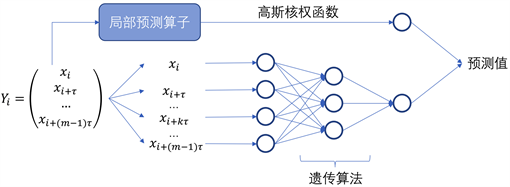Figure 4. Flowchart of hybrid neural network model

6. 预测Table 1. Comparison of prediction result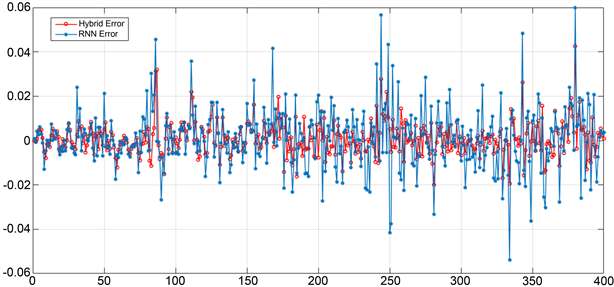Figure 5. Error comparison of hybrid neural network and recurrent neural network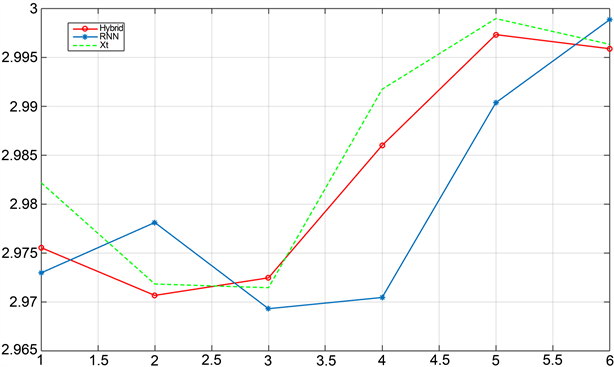Figure 6. Prediction result comparison of hybrid neural network and recurrent neural network

7. 结论

NOTES

*通讯作者。

 罗帅, 等. 风速时间序列混沌特征分析及非线性短期预测[J]. 能源工程, 2019(5): 50-56.

 陈伟, 何家欢, 裴喜平. 基于相空间重构和卷积神经网络的电能质量扰动分类[J]. 电力系统保护与控制, 2018, 46(14): 87-93.

 李巧茹, 等. 基于相空间重构和PSO-GPR的短时交通流预测[J]. 交通信息与安全, 2019, 37(2): 70-76.

 周建中, 彭甜. 长江上游径流混沌动力特性及其集成预测研究[J]. 长江科学院院报, 2018, 35(10): 1-9.

 黄飞江, 等. 基于灰色模型和混沌时间序列的卫星钟差预测算法[J]. 电子学报, 2019, 47(7): 1416-1424.

 高红兵, 潘瑾, 陈宏民. 我国证券市场混沌的判据[J]. 系统工程, 2000, 18(6): 28-32.

 尹海员, 华亦朴. 我国股票市场流动性的非线性动力学特征研究: 基于分形理论的检验[J]. 管理评论, 2017, 29(8): 43-52.

 刘超. 证券投资基金系统的非线性实证研究[J]. 管理评论, 2011, 23(12): 18-25.

 沈菲. 经济系统中的随机分岔与混沌现象研究[D]: [博士学位论文]. 天津: 天津大学, 2005.

 卢山. 基于非线性动力学的金融时间序列预测技术研究[D]: [博士学位论文]. 南京: 东南大学, 2006.

 顾秋宇, 朱捷, 徐晶. 混沌时间序列在股票价格长期预测中的应用[J]. 哈尔滨商业大学学报(自然科学版), 2015, 31(1): 108-111+124.

 王维, 范彦伟. 基于混沌神经网络算法的CPI预测[J]. 统计与决策, 2012(10): 22-24.

 李哲敏, 许世卫, 崔利国, 张建华. 基于动态混沌神经网络的预测研究——以马铃薯时间序列价格为例[J]. 系统工程理论与实践, 2015, 35(8): 2083-2091.

 韩慧婧. 多尺度下长记忆金融混沌序列预测研究[D]: [硕士学位论文]. 太原: 山西财经大学, 2018.

 梁春文. 中国与欧盟碳排放权交易价格波动比较研究[D]: [硕士学位论文]. 广州: 暨南大学, 2018.

 Chen, P. (1998) Empirical and Theoretical Evidence of Economic Chaos. System Dynamics Review, 4, 81-108.
https://doi.org/10.1002/sdr.4260040106

 Takens, F. (1981) Dynamic Systems in Turbulence. In: Rand, D. and Young, L.S., Eds., Dynamical Systems and Turbulence, Warwick 1980. Lecture Notes in Mathematics, Springer, Berlin, Heidelberg, 366-381.
https://doi.org/10.1007/BFb0091924

 ProcacciaI, G.P. (1983) Measuring the Strangeness of Strange Attractors. Physica D: Nonlinear Phenomena, 9, 180-208.
https://doi.org/10.1016/0167-2789(83)90298-1

Top